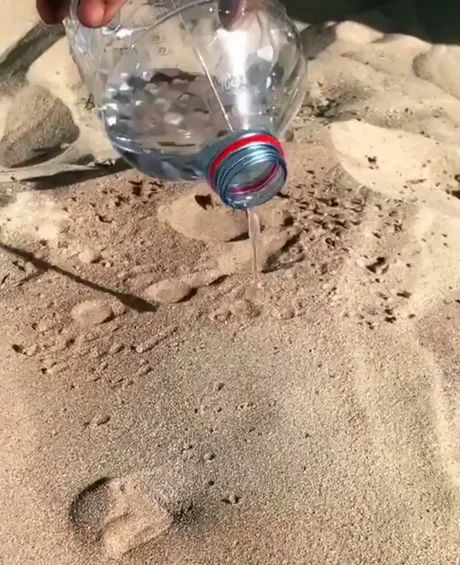# 外國人說「把水倒入沙裡」是在比喻什麼？Image source: https://9gag.com/gag/a8oxOvp

One recent (((最近的))) weekend (((周末))) I had my family (((家人))) out for a beach (((沙灘))) day (((日子))) in Northern California (((北加州))) , and watched (((觀看))) in puzzlement (((困惑))) as (((正當))) my grandson (((孫子))) and his friends (((朋友))) tried (((試著))) to fill (((填滿))) a moat (((護城河))) with (((透過))) water for their sand castle (((城堡))) .

One of the older (((年紀較大的))) children (((孩子))) kept (((持續))) encouraging (((鼓勵))) the younger (((年紀較小的))) kids (((孩子))) to “get (((取來))) more water” and “go faster (((更快的))) bringing (((帶來))) buckets (((水桶))) from sea (((海))) to sand, but inevitably (((無可避免地))) the water in their moat would seep (((滲入))) away (((離開))) , and finally (((最終))) in frustration (((沮喪))) with the effort (((努力))) they all just stomped (((踩踏))) down the castle and moved onto (((移轉至))) more interesting (((有趣的))) and rewarding (((有回報的))) activities (((活動))) , like (((像是))) chasing (((追逐))) waves (((波浪))) .

## pour water on sand (pɔr ˈwɔtər ɑn sænd) / pour water into sand (pɔr ˈwɔtər ˈɪntu sænd)

pour是傾倒，sand是沙子。pour water on sand是在沙子上倒水；pour water into sand是把水倒進沙裡，兩者意思是一樣的。從前述兩個例子，我們可以推理並聯想，你把水倒在沙灘的乾沙堆上，水只會不斷被吸走，倒再多都沒用，是不會看到一攤小水池的。所以把水到入沙裡就是在「做白工」，做無謂且無意義的事。

Approving (((核准))) a vaccine (((疫苗))) for the Covid-19 virus (((病毒))) too (((太過))) soon (((很快的))) without (((沒有))) sufficient (((足夠的))) test (((測試))) results (((結果))) will be just like (((像是))) pouring water on sand, and even (((甚至))) kill (((殺死))) people (((人們))) in worst (((最糟的))) scenarios (((情節、狀況))) .

Don’t waste (((浪費))) your time on this, or you’ll be pouring water into sand.

## 籠 (かご)で水 (みず)を汲 (く)む / 無駄骨 (むだぼね)を折 (お)る

こんな緊急事態 (きんきゅうじたい) になってはもう (かご) 水を汲 (みずをく) むようなことはするんじゃない！もっと (やく) ()つことをしてください。

👉

(かれ) 説得 (せっとく) しようとしても無駄骨 (むだぼね) () るだけよ。 (かれ) (けっ) して心変 (こころが) わりしないでしょうから。

👉